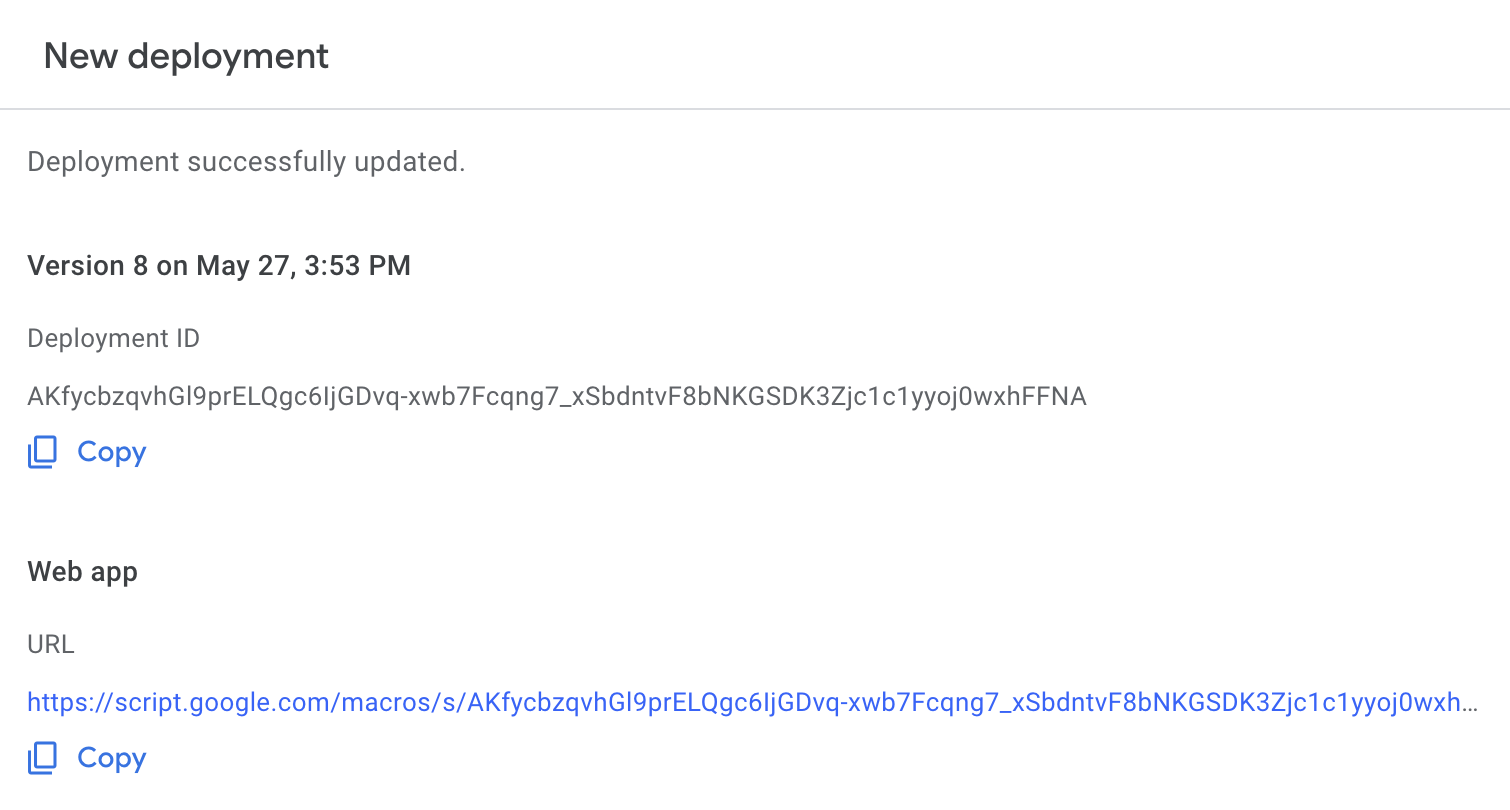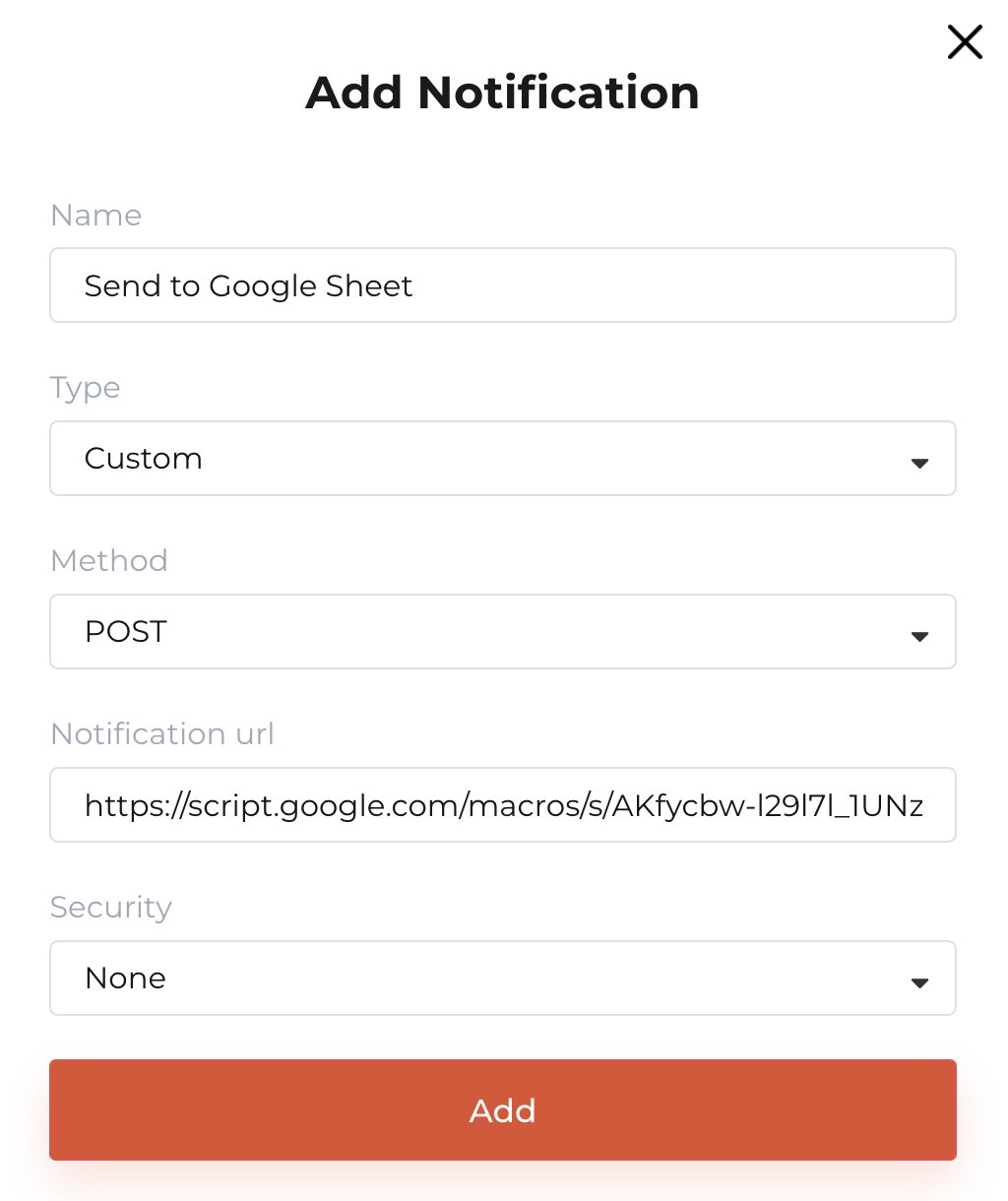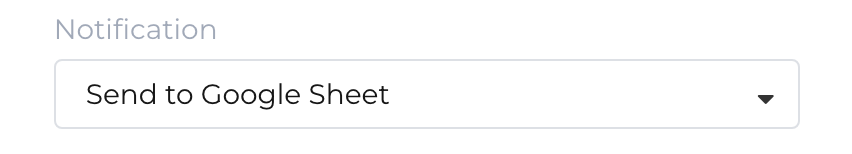It is simple to configure NoahFace to forward user events to a Google Sheet. To do this, complete the following steps: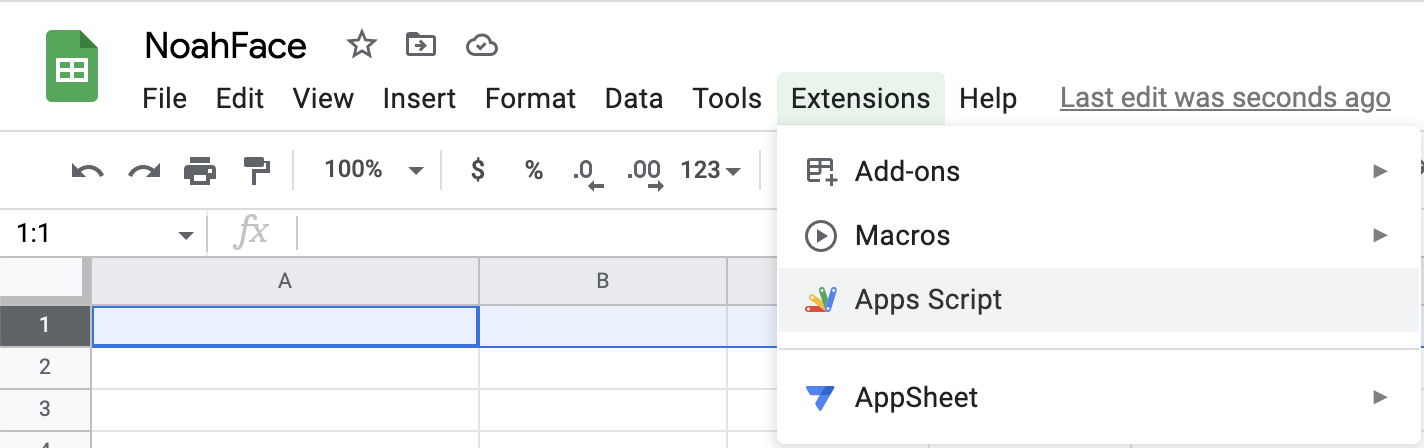2. Create a script with a doPost() function which extracts and processes the event properties. For example:

```function doPost(http) {
var event = JSON.parse(http.postData.contents);
var lastRow = Math.max(sheet.getLastRow(),1);
sheet.insertRowAfter(lastRow);
sheet.getRange(lastRow + 1, 1).setValue(event.time);
sheet.getRange(lastRow + 1, 2).setValue(event.number);
sheet.getRange(lastRow + 1, 3).setValue(event.firstname);
sheet.getRange(lastRow + 1, 4).setValue(event.lastname);
sheet.getRange(lastRow + 1, 5).setValue(event.org);
sheet.getRange(lastRow + 1, 6).setValue(event.site);
sheet.getRange(lastRow + 1, 7).setValue(event.device);
sheet.getRange(lastRow + 1, 8).setValue(event.type);
sheet.getRange(lastRow + 1, 9).setValue(event.detail);
return HtmlService.createHtmlOutput("Success");
}
```

3. Select Deploy / New Deployment, provide a Description, provide Access to "Anyone", and select Deploy: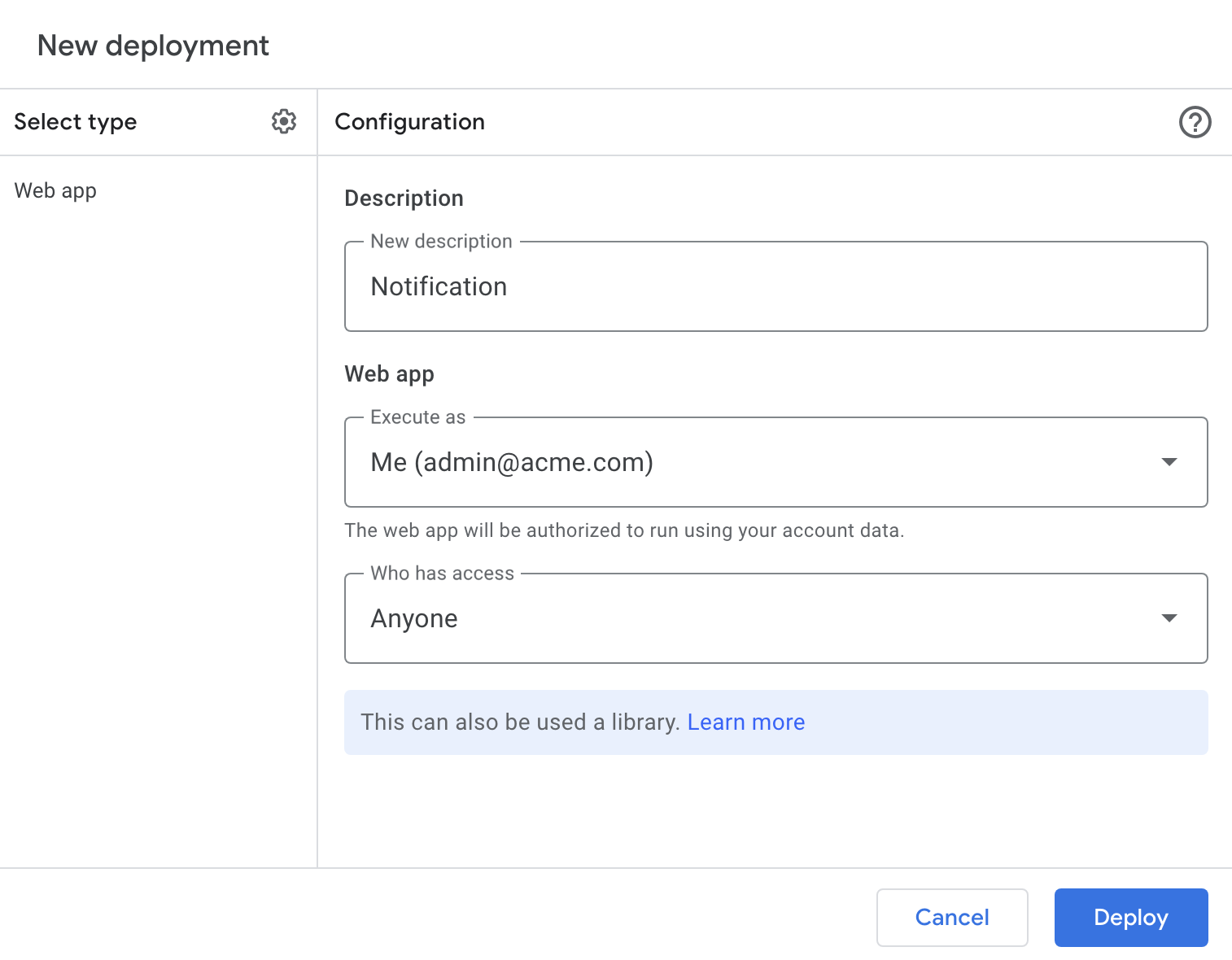4. Copy the Web app URL: